Theory & Practice - Issue 01 - Collision Detection by (17 May 2000) Return to The Archives
 IntroductionAh, collision detection. It's an important part of many games. It's there in Pacman, in Pong, and it's in Quake 3. Collision detection can be used for physics, for restricting movement, for determining whether a weapon or bad guy affects a player, etc. Collision detection methods vary depending on what's required, but there are some general principles that are useful for many types of games. Today, I am going to talk about some of these.

 In GeneralBasically collision detection is a system of determining whether two objects collide. If there are more than two objects, then you can consider pairs of objects individually. What constitutes an "object" varies with implementations.Most collision detection methods usually deal with temporary positions reflecting where the object(s) are about to be. If a collision occurs with the temporary positions, the movement of the objects could be reconsidered. Perhaps they shouldn't move at all. Perhaps they should move just enough to touch each other. Usually what happens is: 1) Future Position Calculation 2) Collision Detection 3) Collision Handling

 Do They Intersect?In collision detection you usually want to know whether two objects intersect. When we say "collide" we usually assume movement in time. If we have a still picture, then we can say "intersect." Let's consider the simplest case: we have two line segments in 1 dimension, and we need to know whether or not they overlap (Figure 1).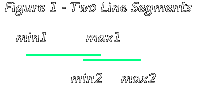These two segments intersect if min1

 Collision in TimeSometimes you'll want to work with more than simply hypothetical future positions in order to determine whether a collision occurred. This happens when your objects are zipping along, several units of length at a time, and might pass through something briefly, but not enough to wind up in it. Consider the following picture: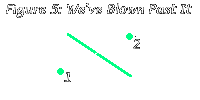Suppose if your object was as small as the circle in the picture, and it moved 10 units each frame. Eventually you might realize that the object is now on the other side of the wall. What you want is to predict, while the object is in position 1, whether the object will go through the wall if it's moved 10 units, and if so, where exactly the object will reach the wall.

Instead of obtaining a solution to this particular problem (with the circle and the wall), I will present some general methods for solving these kinds of problems. This is where mathematical thinking begins to really help.

Think of the prediction problem as a problem in time. Two objects are moving relative to one another. Their positions are functions of time. You want to know exactly when something happens -- for example if they collide. If you find the time that happens, you can find their positions and anything else that depends on time.

What you want is to define some sort of relationship between the two objects that changes as a function of time. Here's what this kind of prediction problem would involve. We have:

 P1(t), P2(t) Positions P1 and P2 of the two objects as functions of time. (This can be in 1, 2, 3, etc. dimensions) R(t) The relationship between positions P1 and P2. Since both P1 and P2 are functions of time, R(P1(t), P2(t)) can just depend on time. n Some value that you want the relationship to be.

If you're a bit confused by the generality of these functions, it's okay. Basically it's like this:

The position functions can be anything you want. If an object is moving in a straight line, then you have a linear equation. If you have acceleration, such as gravity, then you might have a quadratic or cubic equation, etc.

Now you want to express the relationship you want in terms of the positions of the objects, and the value you want it to be. If you want two circles to collide, then R(P1, P2) would be the distance between P1 and P2, squared, and n would be the sum of the radii, squared. Now that you have expressed the collision conditions in terms of the object positions, and the object positions in terms of t, then you can express the entire collision scheme (the conditions) in terms of t. What you get is an equation that corresponds to: R(t) = n

Once you have that, you can usually solve for t, because R(t) is usually a polynomial. Thus you get the time at which the collision occurs. It doesn't even have to be collision, it can be some other event. Up until now you do everything on paper. What you finally put into your program is one, optimized line: the equation for t. It looks something like: t = . . .

Still confused? Let's take a look at some examples.

 Example 1
We have two circles, with centers (x1,y1), (x2,y2) and radii r1, r2. The radii remain constant, but the first circle moves (2, 7) in one second, and the other moves (-1, 2) in one second. The question is, will they collide in that second?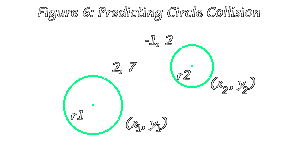Solution
From this we have:
P1(t) = (x1 + 2t, y1 + 7t)
P2(t) = (x2 - t, y2 + 2t)
Further,
R(t) = [(x1 + 2t) - (x2 - 2t)]2 + [(y1 + 2t) - (y2 + t)]2 = (x1 - x2 + 3t)2 + (y1 - y2 + 5t)2 =
(x1 - x2)2 + (y1 - y2)2 + 3t(2x1 - 2x2 + 3t) + 5t(2y1 - 2y2 + 5t) =
[32+52]t2 + 2[3(x1 - x2) + 5(y1 - y2)]t + [(x1 - x2)2 + (y1 - y2)2]

This is a quadratic equation in t. We don't even have to solve it for t, because the original question was whether the two circles will collide. So we find the minimum of the function R(t). To do that, we take the derivative of R(t). We get:

R'(t) = 2[32+52]t + 2[3(x1 - x2) + 5(y1 - y2)]

And we solve for t1 when R'(t1) = 0 :

t1 = -[3(x1 - x2) + 5(y1 - y2)] / [32+52]

It is at this point in time that the distance is least between the two centers of the circles. You can visualize this: if the circles start out moving towards each other, for example, over time the distance will decrease and then increase steadily (Figure 7).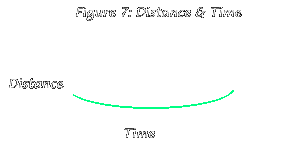We're almost done. First, we see if the distance between the circle centers (squared) at the time t gotten above is less than the sum of the radii (squared). In other words, we check: R(t1) < (r1 + r2)2 ?

If the distance between the centers smaller than the sum of the radii at that t, this means the circles collide at that t. Finally, we have to check whether 0 < t < 1. If the question asked, "will the circles ever collide if they continue moving this way forever?" we would be done. But the question asked whether the circles would "collide in that second." Checking whether t is between 0 and 1 is easy. So, if we've gotten this far, then the circles will collide.

Actually, if we analyze the method we used to get the answer, we can get a general solution to the problem of two moving circles. If circle 1 moves (a1, b1) units per second, and circle 2 moves (a2, a2) units per second, then the minimum distance time will be:

(1) t1 = -[(a1 - a2)(x1 - x2) + (b1 - b2)(y1 - y2)] / [(a1 - a2)2 + (b1 - b2)2]

In our example, a1 - a2 = 3 and b1 - b2 = 5.
In fact, we see that we don't need to know the movements of the circles in absolute coordinates. We only need to know the movement of the balls relative to each other. If we take the center of circle 1 to be the origin, then the equation for t1 can be something like:

(2) t1 = -[ax + by] / [a2 + b2]

Compare this to equation (1).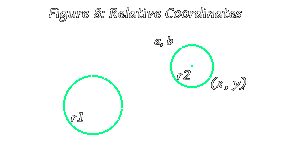Example 2
We have a circle and a line segment going through it. The circle is centered at (a, b) with radius r. The line segment goes from (x1, y1) to (x2, y2). Does the line segment intersect the circle?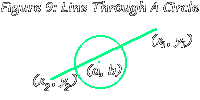Solution
Again, we can consider this a time problem. Let's say the line segment is really a point traveling through time. We have to get the time where the point is closest to the center of the circle, and see whether at that time the distance from the point to the circle (squared) is less than the radius of the circle (squared).

P1(t) = (a, b)
P2(t) = ( x1 + [x2 - x1]t, y1 + [y2 - y1]t ) -- where t goes from 0 to 1

In fact, let's use the work we've already done in Example 1. Let's consider the point to be a circle with radius 0. In that case we make use of equation (2).

t1 = -[(0 - [x2 - x1])(a - x1) + (0 - [y2 - y1])(b - y2)] / [(0 - [x2 - x1])2 + (0 - [y2 - y1])2] =
[(x2 - x1)(a - x1) + (y2 - y1)(b - y2)] / [(x2 - x1)2 + (y2 - y1])2]

That's it. Solve for t1, and see if it's between 0 and 1. If it is, plug it into R(t) [which is the distance between P1(t) and P2(t), squared] and see if it's less than the radius squared.

By the way, if t1 isn't between 0 and 1, that means that an infinite line would intersect that circle, but not the line segment, which runs from (x1, y1) to (x2, y2)

Also, if the distances were relative to the center of the circle, then everything would be shorter to write. Say the circle is centered at (0, 0), has radius r, and the line segment runs from (x1, y1) to (x2, y2).

t1 = -[(x2 - x1)x1 + (y2 - y1)y1] / [(x2 - x1)2 + (y2 - y1)2]

Note: in this particular case there are easier ways of solving the problem. To find the minimum distance of a line AB to a point C, one could take a random point D on the line, and project CD onto the line perpendicular to AB. The absolute value of the resulting vector would be the minimum distance. This example is just to illustrate the general method of predicting collisions.

 Example 3
One more example. This time with bounding boxes. We have a dot that travels from (x1, y1) to (x2, y2) in one second. We have a box with its center at (a, b), width w and height h. Does the dot go through the box? If so, when is it inside the box?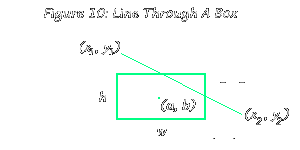Solution
This time we'll set things up a bit differently.

P1(t) = (a, b)
P2(t) = ( x1 + [x2 - x1]t, y1 + [y2 - y1]t ) -- where t goes from 0 to 1

We'll have two relationship functions: the horizontal distance from the center of the box and the vertical distance.

R1(t) = abs( x1 + [x2 - x1]t - a ) -- the horizontal distance
R2(t) = abs( y1 + [y2 - y1]t - b ) -- the vertical distance

This time we take no derivatives. Due to the question, we need to find when the dot enters the box and when it leaves. So we solve for t in the following equations:

R1(t) = w/2
R2(t) = h/2

For the first equation we get two values for t. This is because of the absolute value operation in R1(t).

t1 = (w/2 - x1 + a) / (x2 - x1)
t2 = -(w/2 + x1 - a) / (x2 - x1)

This is expected. As the dot travels along the line, it will "enter" and "leave" the horizontal interval of the box. And of course, the path of the dot cannot be vertical, since then the point would make no horizontal progress. This is demonstrated by the fact that, on a vertical line, (x2 - x1)=0 and dividing by zero is not allowed.
Similarly, for vertical distance,

t3 = (h/2 - y1 + b) / (y2 - y1)
t4 = -(h/2 + y1 - b) / (y2 - y1)

So we have found the times when the dot "enters" and "leaves" the vertical interval of the box. (The path of the dot cannot be horizontal, for the same reasons as stated above.)

We should, as before, check whether t is between 0 and 1. All that remains after that is to see whether the time intervals (t1, t2) and (t3, t4) overlap. This can be done using everyone's favorite intersection lemma:

If t14 and t32 then the dot enters the box for a period of time on its way from (x1, y1) to (x2, y2).

Finally, we can get the start and end of this time period. To do that, we sort t1, t2, t3 and t4 in order from earliest to latest (or vice versa), and the middle two are the beginning and end of the time period the dot is inside the box.
So, what you do really depends on what you're looking for. If you want to find out when the objects begin to collide, then you solve R(t) = n for t. (You might get more than one t depending on whether R(t) is linear, quadratic, etc.) If you just want to find out whether the objects will collide in the future, you can find out when R(t) is at its minimum by solving for t in R'(t) = 0 and only check that 'minimum' case. The derivative of R(t) is simpler than R(t); for example, if R(t) is quadratic in t then R'(t) is linear.

 Summing Up So FarHere ends the first part on collision detection. We went rather quickly, but I hope you're left with some general ideas in your mind regarding general principles of collision detection. Perhaps they will help you implement the specialized collision detection you'll need in your projects.A note: There was quite a bit of math in this discussion, but don't be put off by that fact. You don't usually have to understand all the math to grasp the underlying idea. Usually, the math in these discussions won't be very involved, and I will talk more about programming techniques. However, understanding the math behind these techniques provides a good foundation. I have found that, often, math comes in very handy in solving problems of this sort; it serves to organize your thoughts and illustrate the relationships between different qualities.Next time, I will explore some common collision detection situations involving polygons, and the methods of solving them. Please drop me a line, and tell me what you thought. And stay tuned for the next issue :)

 Links And AcknowledgementsHere's an article on Gamasutra by Jeff Lander, entitled "When Two Hearts Collide..." (Bounding Boxes) This article, by Nick Bobic, talks about some advanced topics in collision detection, such as hierarchy trees.